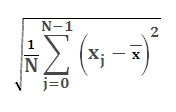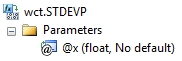# SQL Server population standard deviation function

STDEVP

Updated: 28 February 2011

Note: This documentation is for the SQL2008 (and later) version of this XLeratorDB function, it is not compatible with SQL Server 2005.

Use the aggregate function STDEVP to return the standard deviation for an entire population. This function differs from the built-in SQL Server STDEVP function in that it employs a two-pass and more numerically stable method for calculating the population standard deviation and under certain conditions will return a different value than the built-in function.

The standard deviation is a measure of how widely values are dispersed from the mean. The equation for STDEVP is:SyntaxArguments
@x
the values used in thepopulation standard deviation calculation. @ x is an expression of type float or of a type that can be implicitly converted to float.
Return Types
float
Remarks
·         If you want measure the standard deviation for a sample, then use the STDEV function.
·         NULL values are not included in the standard deviation calculation.
·         STDEVP is an aggregate function and follows the same conventions as all other aggregate functions in SQL Server.
·         If you have previously used the STDEVP scalar function, the STDEVP aggregate has a different syntax. The STDEVP scalar function is no longer available in XLeratorDB/statistics2008, though you can still use the scalar STDEVP _q
Examples
SELECT wct.STDEVP(x) as STDEVP
FROM (VALUES
(91.3698),
(76.3382),
(74.5692),
(85.2957),
(99.0112),
(86.99),
(70.7837),
(72.834),
(78.1644),
(77.7472),
(66.0627),
(59.781),
(68.4793),
(78.6103),
(59.8621)
) n(x)

This produces the following result.
STDEVP
----------------------
10.6563315803558

(1 row(s) affected)

In the following example, we will use demonstrate the difference between the SQL Server STDEVP calculation and XLeratorDB STDEVP calculation. Using the XLeratorDB/math SeriesFloat function to create a million rows of randomly generated values between 1,000,000,000 and 1,000,000,010
SELECT ROUND(SeriesValue * 10, 0) + 1000000000 as x
INTO #n
FROM wctMath.wct.SERIESFLOAT(0,1,'',1000000,'R')

SELECT STDEVP(x) as [SQL SERVER STDEVP]
,wct.STDEVP(x) as [XLDB STDEVP]
,STDEVP(x-1000000000) as [SQL SERVER STDEVP adjusted]
FROM #n

DROP TABLE #n

This produces the following result. Your results will vary, since the dataset is randomly generated. The first column shows the built-in SQL Server STDEVP calculation, the second shows the XLeratorDB calculation and the third column shows the built-in calculation for x minus 1,000,000,000.
SQL SERVER STDEVP            XLDB STDEVP SQL SERVER STDEVP adjusted
---------------------- ---------------------- --------------------------
513.945997848023       2.91608615295645           2.91608615296582

(1 row(s) affected)

### SupportCopyright 2008-2023 Westclintech LLC         Privacy Policy        Terms of Service